# TPLinker: Single-stage Joint Extraction of Entities and Relations Through Token Pair Linking

## Basic Idea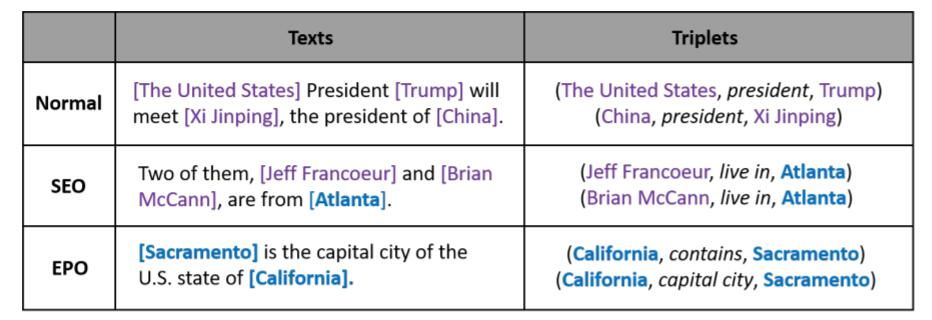• EPO(Entity Pair Overlap): 实体对重叠是指实体对之间具有多种关系, 这些三元组共享相同的实体.
• SEO(Single Entity Overlap): 单实体重叠是指有单个实体被多个三元组共享.

### HandShaking Tagging Scheme

#### Tagging

• EH-to-ET: 两Token分别为同实体起止位置(Entity Head to Entity Tail).

• ST-to-OT: 两Token分别为Subject和Object的结束位置(Subject Tail to Object Tail).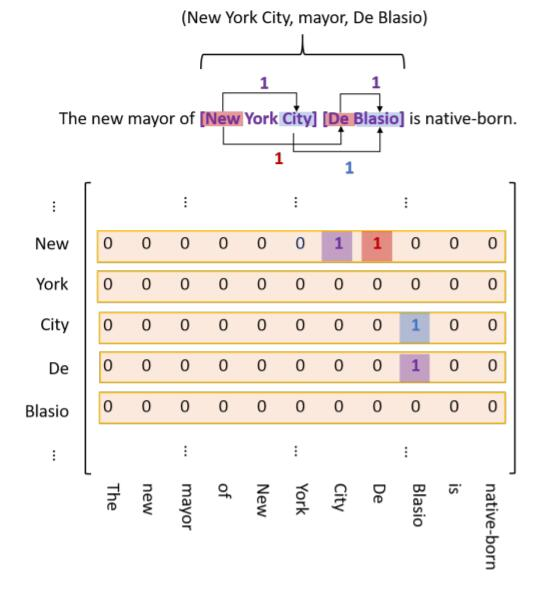• EH-to-ET: 图中的紫色Tag, (New, City) 就分别是New York City的头尾, (De, Blasio) 分别是De Blasio 的头尾.
• SH-to-OH: 图中的红色Tag, (New, De) 分别是给定关系New York CityDe Blasio 的头.
• ST-to-OT: 图中的蓝色Tag, (City, Blasio) 分别是给定关系New York CityDe Blasio 的尾.

SubjectEH-to-ETSH-to-OHST-to-OT
New York(New, York)(New, De)(York, Blasio)
New York City(New, City)(New, De)(City, Blasio)

1. 若按图中方式建模, 矩阵会非常稀疏. 并且因为实体的尾永远都在头的前面.
3. 有趣的是, Object可能出现在Subject前面, 所以对于SH-to-OHST-to-OT这两种链接类型, 直接把下三角丢掉是错误的.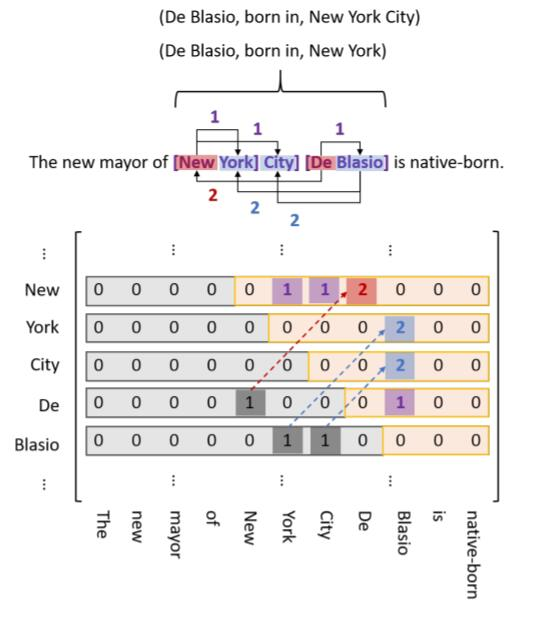#### Decoding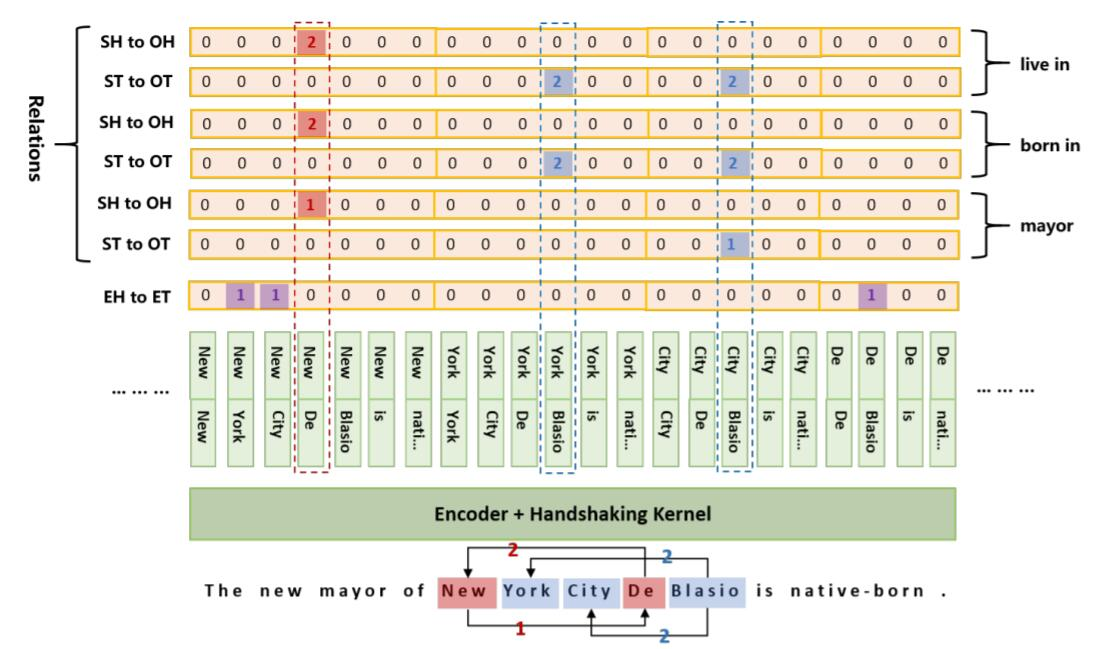EH-to-ET分别有三个Token Pair, (New, York), (New, City), (De, Blasio), 分别对应实体New York, New York City, De Blasio, 这是区分是否为同实体的Tag, 必须要与其他两种Link Type结合使用.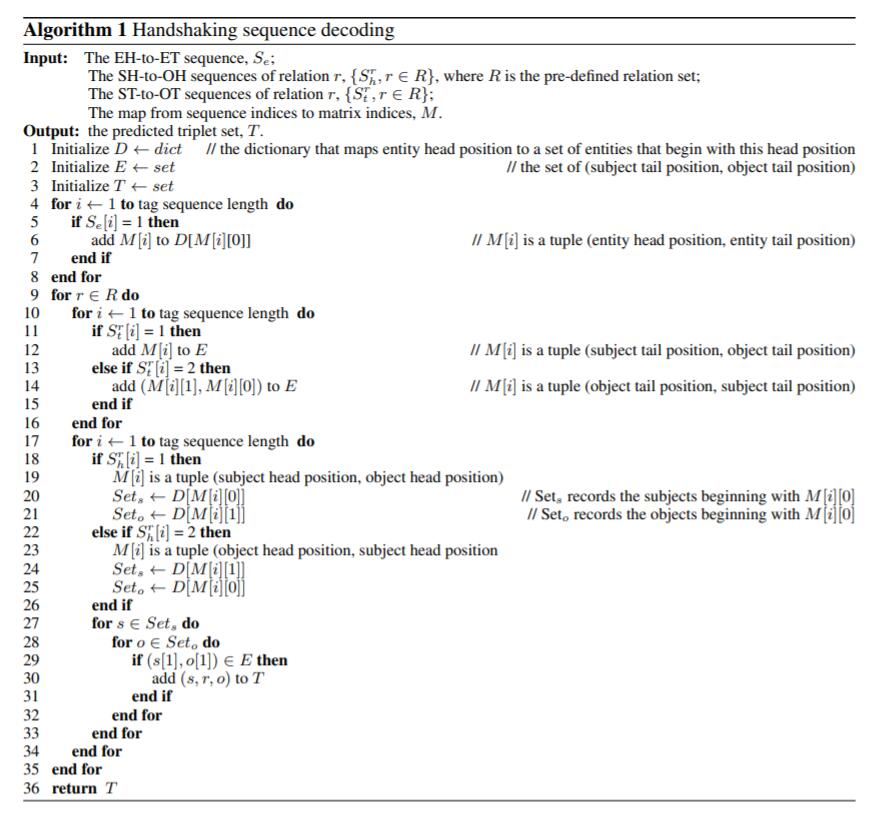1. 根据EH-toET的表, 检测所有存在的实体, 存入字典$D$.
2. 对每一种关系, 根据ST-to-OT的表记录Subject和Object的尾位置存入集合$E$. 将Tag为1的顺序记下来, 及Tag为2的逆序记下来.
3. 对每一种关系, 根据SH-to-OH的表获得Subject和Object的头位置(Tag为1, 2, 同上), 直接去$D$ 中查询对应的实体对, 查询到后去$E$ 中做匹配, 如果命中, 则抽取出三元组.
4. 对每一种关系, 根据SH-to-OH的表,

### Token Pair Representation

$$\mathbf{h}_{i, j}=\tanh \left(\mathbf{W}_{h} \cdot\left[\mathbf{h}_{\mathbf{i}} ; \mathbf{h}_{\mathbf{j}}\right]+\mathbf{b}_{h}\right), j \geq i$$

### Handshaking Tagger

\begin{aligned} &P\left(y_{i, j}\right)=\operatorname{Softmax}\left(\mathbf{W}_{o} \cdot \mathbf{h}_{i, j}+\mathbf{b}_{o}\right) \\ &\operatorname{link}\left(w_{i}, w_{j}\right)=\arg \max _{l} P\left(y_{i, j}=l\right) \end{aligned}

### Loss Function

$$L_{l i n k}=-\frac{1}{N} \sum_{i=1, j \geq i}^{N} \sum_{\ast \in\{E, H, T\}} \log P\left(y_{i, j}^{\ast}=\hat{l}^{\ast}\right)$$

$N$ 为句子长, $\hat{l}$ 为正确Tag, $E, H, T$ 分别代表EH-to-ET, SH-toOH, ST-to-OT.

## Experiments

### Dataset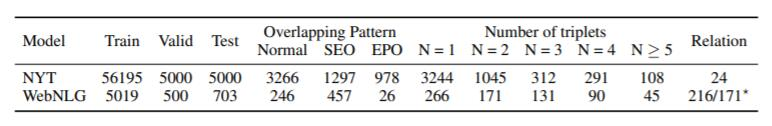### Main Results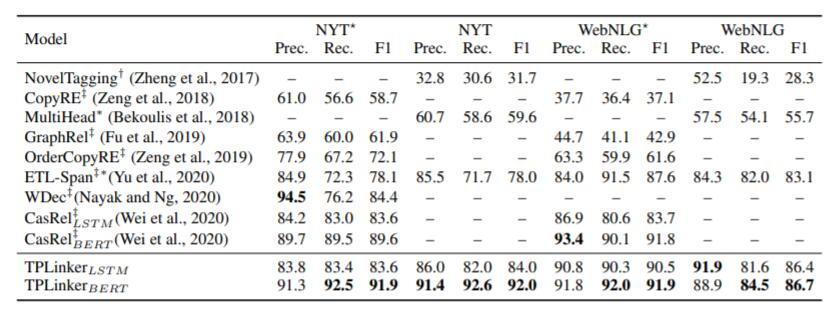### Analysis on Different Sentence Types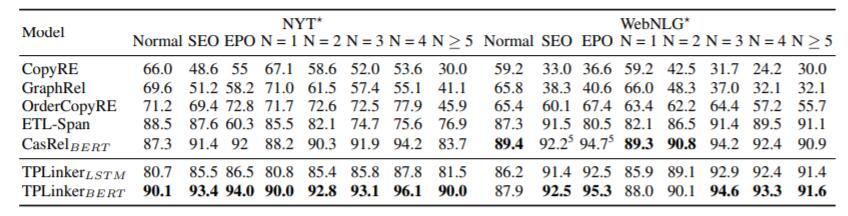### Analysis on Computational Efficiency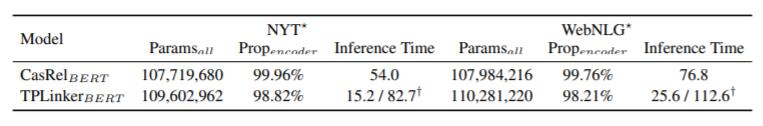## Summary

上一篇CasRel: A Novel Cascade Binary Tagging Framework for Relational Triple Extraction
A Novel Cascade Binary Tagging Framework for Relational Triple Extraction本文是论文A Novel Cascade Binary Tagging Framework f
2021-10-04
目录